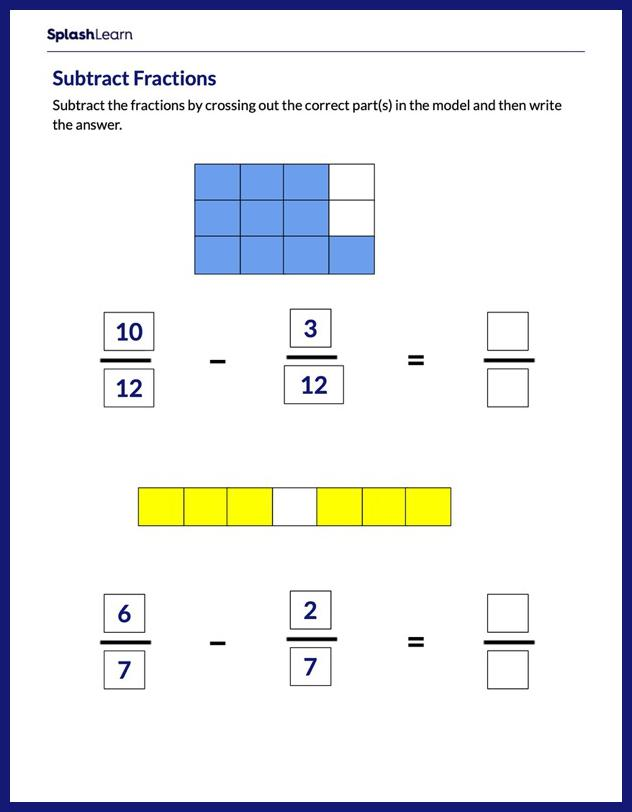# Subtracting Fractions Using Visual Model Worksheet

Home > Subtracting Fractions Using Visual ModelStruggles with subtraction of fractions can easily be overcome if students practice the concept in a fun and engaging way! The worksheet encourages students to use fraction models as tool and develop a solid understanding of subtraction of fractions. Here the rigor is beautifully balanced by asking students to work with fractions with like denominators.